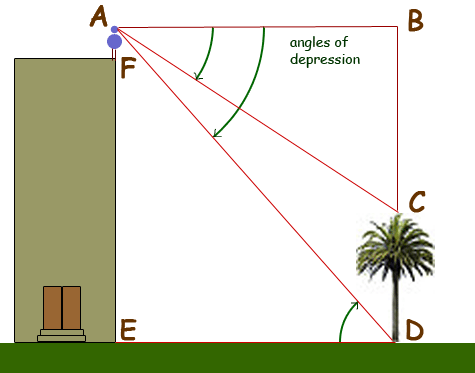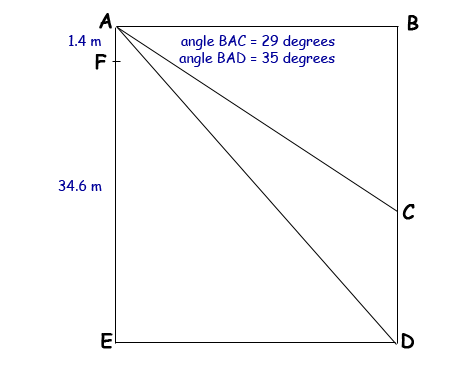SEARCH HOMEMath Central Quandaries & QueriesQuestion from romaine, a student: a woman of height 1.4m standing on top of a building of height 34.6m veiws a tree some distance away. she observes that the angle of depression of the bottom of the tree is 35 degrees, and the angle of depression of the top of the tree is 29 degrees. assume that the building and the tree is on level ground. (a) calculate the distance of the woman from the top of the tree measured along her line of sight. (b) determine the height of the tree. if u can send the working out please do thank you.Hi Romaine.

I've made a drawing and added some lines and labelled some corners:We can turn this into a simpler geometrical diagram as follows:Here are the steps you can use to solve your problem:

1. Find the length of BD.
2. Find the length of AB using trigonometry for the triangle BAD.
3. Find the length of AC using trigonometry for the triangle BAC.
4. Find the length of BC using trigonometry or Pythagorus Theorem.
5. Find the length of CD.

Hope this helps,
Stephen La Rocque.

PS: We are making the assumptions that her height is measured at eye level (or that this is "close enough") and that both the building and the tree are straight upright. In Pisa, Italy, your results may differ.Math Central is supported by the University of Regina and The Pacific Institute for the Mathematical Sciences.